# 论文引入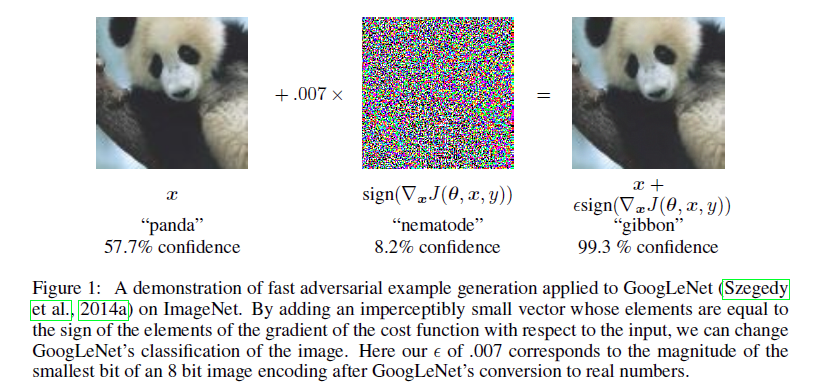Goodfellow认为对于简单的线性模型(x;y)，x为真实样本，y为对应的标签，在对x的每个维度添加微小扰动的情况下，扰动的每一个维度上都发生了影响，随着维度的增大， 这个扰动很容易导致最后的输出有较大的变化。对于非线性模型神经网络是因为太“线性”而不能抵抗对抗样本，无论LSTMs, ReLUs, 和maxout网络都设计得很“线性”，所以他们很容易被利用。 我们可以想象一个具有高度线性的网络训练出来的函数，它的高阶导数近似于0，Taylor展开以后占主导作用的是线性部分，所以可以类比为线性分类器。 深度模型之所以在对抗样本的无力最主要的还是由于对抗样本其线性部分的存在。

$\begin{equation} L_{adv}(x_l, \theta) := D[q(y \vert x_l), p(y \vert x_l + r_{adv}, \theta)] \end{equation}$

$\begin{equation} r_{adv} := \arg \max_{r;\Vert r \Vert leq \epsilon} D[q(y \vert x_l), p(y \vert x_l + r_{adv}, \theta)] \end{equation}$

$\begin{equation} r_{adv} = \epsilon \frac{g}{\Vert g \Vert_2},where g = \triangledown_{x_l} D[h(y;y_l), p(y \vert x_l, \theta)] \end{equation}$

$\begin{equation} \widetilde{J}(\theta ,x,y) = \alpha J(\theta ,x,y) + (1 - \alpha)J(\theta ,x+ \epsilon sign(\nabla J_x(\theta ,x,y))) \end{equation}$

VAT则是在对抗训练的基础上，提出了LDS(local distributional smoothness)。VAT提出的LDS也可以理解为在原有模型的基础上加上正则项，这个正则项可以实现局部分布平滑， 也就是不单单关注样本下梯度的扰动（同性噪声），而是对梯度周围进行扰动（各向异性噪声）处理，正因为这样处理，VAT可以不仅仅适用于纯监督环境， 也适用于半监督训练。

• VAT在AT的基础上设计了LDS，可以实现局部平滑
• VAT可以实现半监督下模型的对抗训练，并且取得了不错的实验效果
• VAT本身计算上没有太多超参设置，$r_{vadv}$的快速估计提升了计算速度

# VAT介绍

VAT整体的设计思路源于对抗训练，然而对抗训练只适用于监督模型的训练，VAT则实现了半监督学习。我们先来规定一下符号，对于标签数据集记为$\mathcal D_l = {x_l^{(n)},y_l^{(n)} \vert n=1,…,N_l}$ 对应的标签就是$y_l$，对于无标签数据集$\mathcal D_{ul} = {x_{ul}^{(m)} \vert m=1,…,N_{ul}}$，训练模型$p(y \vert x,\epsilon)$ 使用的就是标签数据$\mathcal D_l$和无标签数据$\mathcal D_{ul}$。

$\begin{equation} L_{qadv}(x_\ast,\theta) = D[q(y \vert x_*), p(y \vert x_* + r_{qadv},\theta)] \end{equation}$ $where r_{qadv} := \arg \max_{r;\Vert r \Vert \leq \epsilon} D[q(y \vert x_*), p(y \vert x_*,\theta)]$

\begin{equation} \begin{aligned} & LDS(x_\ast,\theta) := D[p(y \vert x,\hat{\theta}), p(y \vert x_\ast + r_{vadv},\theta)]\\ & r_{vadv} := \arg \max_{r;\Vert r \Vert_2 \leq \epsilon} D[p(y \vert x,\hat{\theta}), p(y \vert x_\ast,\theta)] \end{aligned} \end{equation}

$\begin{equation} \mathcal R_{vadv}(\mathcal D_l,\mathcal D_{ul},\theta) := \frac{1}{N_l,N_{ul}} \sum_{x_* \in \mathcal D_l,\mathcal D_{ul}} LDS(x_*,\theta) \end{equation}$

$\begin{equation} \mathcal l(\mathcal D_l,\theta) + \alpha \mathcal R_{vadv}(\mathcal D_l,\mathcal D_{ul},\theta) \end{equation}$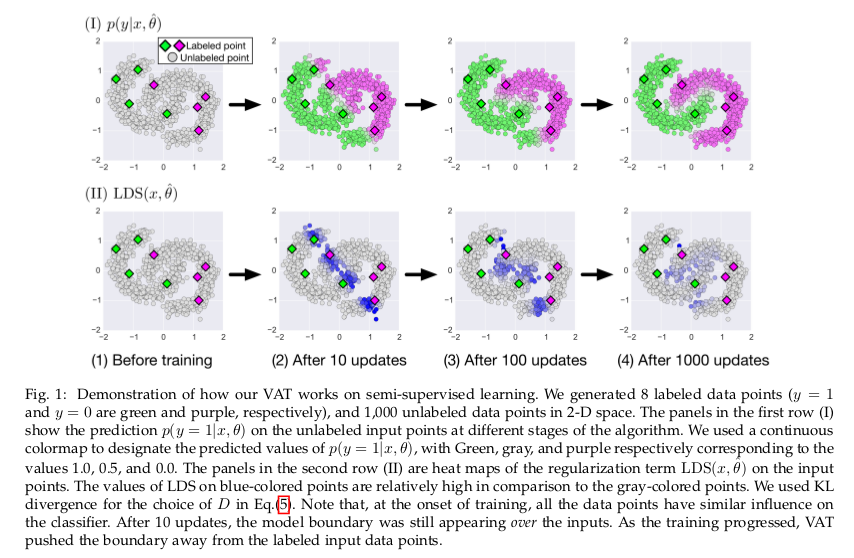# 快速确定$r_{vadv}$值

$\begin{equation} D(r,x,\theta) = f(0) + f'(0)r + \frac{f''(0)}{2!}r^2 ... \approx \frac{1}{2}r^T H(x,\hat{\theta})r \end{equation}$

\begin{equation} \begin{aligned} r_{vadv}(x,\theta) &\approx \arg\max_{r} \{r^TH(x,\theta)r; \Vert r \Vert_2 \leq \epsilon\} \\ &= \epsilon \overline{u(x,\theta)} \end{aligned} \end{equation}

$\begin{equation} d \gets \overline{Hd} \end{equation}$

\begin{equation} \begin{aligned} Hd &\approx \frac{\nabla_r D(r,x,\hat{\theta}) \vert_{r=\xi d} -\nabla_r D(r,x,\hat{\theta})\vert_{r=0}}{\xi} \\ &= \frac{\nabla_r D(r,x,\hat{\theta})\vert_{r=\xi d}}{\xi} \end{aligned} \end{equation}

$\begin{equation} d \leftarrow \overline{\nabla_r D(r,x,\hat{\theta}) \vert_{r=\xi d}} \end{equation}$

\begin{equation} \begin{aligned} &r_{vadv} = \epsilon \frac{g}{\Vert g \Vert_2} \\ &where g = \nabla_r D[p(y \vert x,\hat{\theta}), p(y \vert x+r,\hat{\theta})] \vert_{r = \xi d} \end{aligned} \end{equation}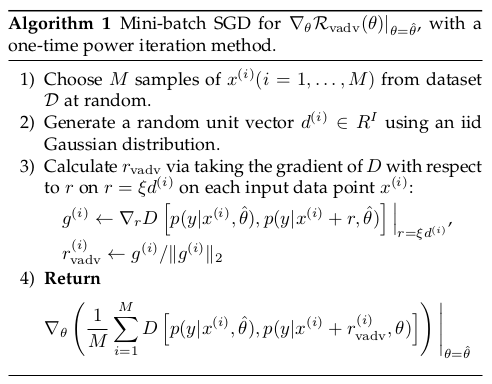$\begin{equation} \mathcal R^{(K)}(\theta ,\mathcal D_l,\mathcal D_{ul}) := \frac{1}{N_l + N_{ul}} \sum_{x \in \mathcal D_l,\mathcal D_{ul}} \mathbb E_{r_K}[D[p(y \vert x,\hat{\theta}), p(y \vert x+r_K,\theta)]] \end{equation}$

VAT平滑模型的方向比RPT平滑模型的方向更具确定性，模型均匀分布在半径$\epsilon$的范围内，RPT学习的稳定性总是受到$\mathcal R^{(0)}$的方差影响。 后面实验中，文章也对RPT和VAT做了进一步的比较。

# VAT实验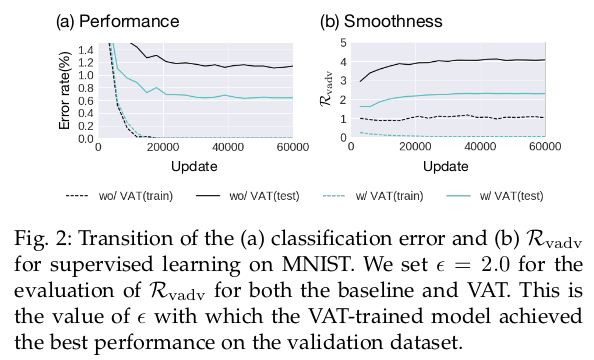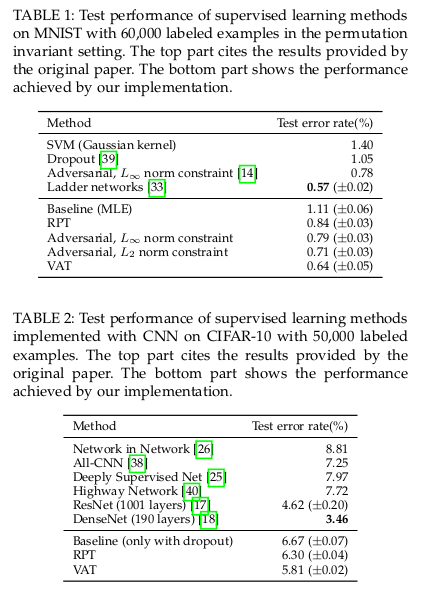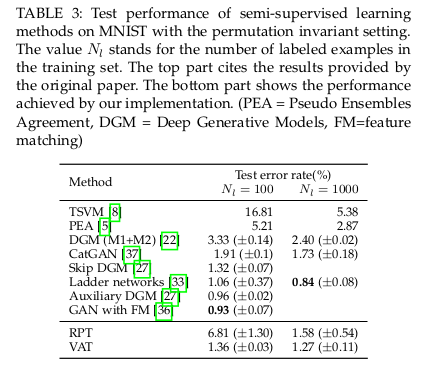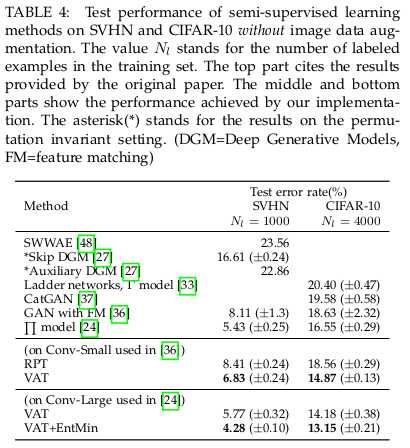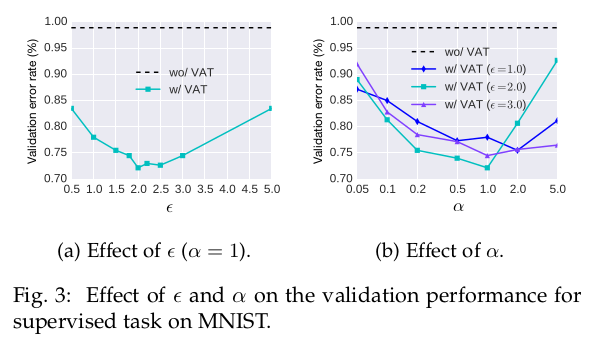VAT较RPT在之前的定量表格下已经展示了优势，为了进一步比较，论文又做了对比实验：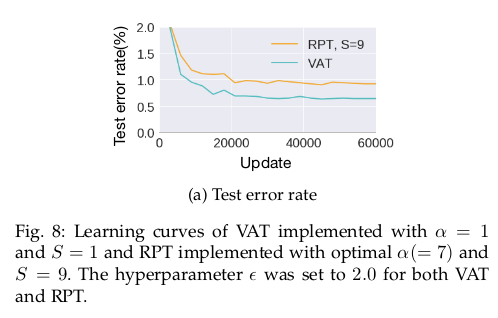# 总结

VAT是在对抗训练的基础上将监督学习模型扩展到半监督学习模型，同时模型在同向噪声鲁棒性提高到可以在异向噪声具有鲁棒性，在监督和半监督条件下都取得不错的实验结果。 快速近似求解$r_{vadv}$，带来了较小的计算成本，模型只有两个超参数。论文实验部分写的很是详细，感兴趣的可以进一步阅读原文了解。